# Designing a wideband analog voltage and current meter. Part 2

## First and second amplifiers and range switch

The schematic is shown in Figure 3.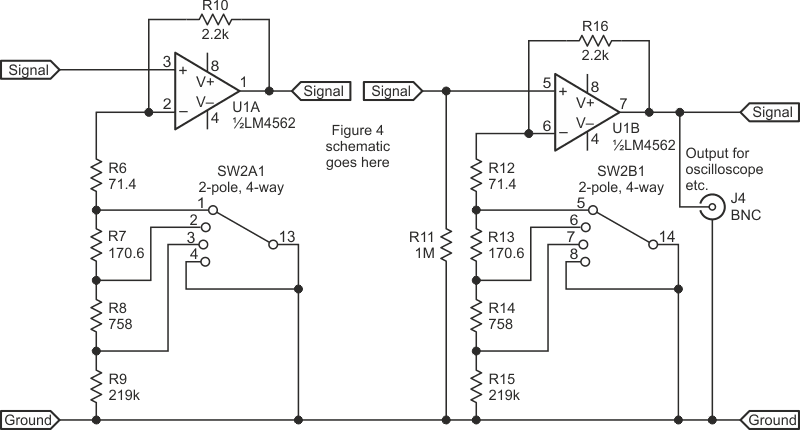Figure 3. First and second amplifiers and range switch.

The switching is arranged so that there are no unused resistors attached to the signal circuit; they are attached to the ground. The switching for the 3/10/13 dB attenuators and ‘External filter’ are covered in the next section.

The switches are shown in the 1 V position. Each step changes the gain by 10 dB on each switch pole, giving 20 dB steps (10 times).

The low value of the feedback resistors R10 and R16 is the key to getting wide bandwidth. They need to be as near 2.2 kΩ as possible, which may mean selecting from several components.

R6 and R12 could be E96 series value 71.5 Ω, or 82 Ω || 560 Ω (|| means ‘in parallel’). R7 and R13 are 180 Ω || 3.3 kΩ. R8 and R14 are 820 Ω || 10 kΩ. Other combinations are obviously possible. Additionally, R9 and R15 can be 220 kΩ.

It is necessary to get as close to the required values as possible because the instrument's accuracy depends on it. Preset resistors should be avoided, if possible, or high-quality components should be used; otherwise, calibration stability will suffer.

Note that using the 2.2 kΩ and 220 kΩ resistors for feedback gain setting produces a non-inverting amplifier with a gain of 1.01 (0.086 dB), which is close enough to 1.

## Additional attenuators and external filter connectors

The 3 dB (peak-to-RMS sine wave) and 10 dB (scale magnifier) attenuators, and the external filter switches, are shown in Figure 4.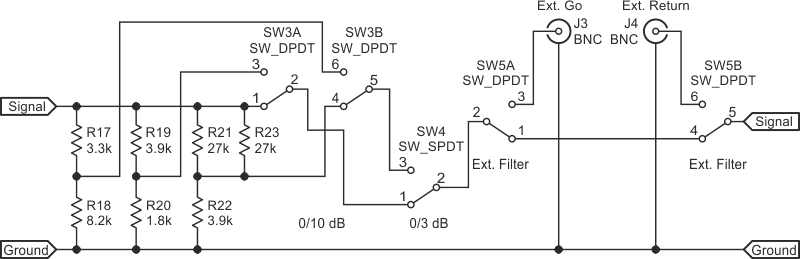Figure 4. Diagram of 3/10/13 dB attenuators and external filter connectors.

As before, it is necessary to measure the resistors and choose those closest to the required values. It helps that, in this case, E12 preferred values can be used at the cost of one extra resistor for the –13 dB network.

It’s useful to examine how well the E12 series resistors give the required attenuation. Table 1 shows the results.

Table 1. Error as a function of attenuation ratio.
 Attenuation db Attenuation ratio Upper arm resistor Lower arm resistor Ratio lower/ (lower + upper) Error % Error dB 3 0.7071 3.3k 8.2k 0.7130 -0.84 -0.062 10 0.3162 3.9k 1.8k 0.3158 0.14 0.012 13 0.2236 13.5k 3.9k 0.2241 –0.24 0.021 3 dB fix 0.7071 3.382k 8.2k 0.7080 –0.12 –0.011

Using measured-value resistors, the error can be minimized by adding a low-value resistor in series with whichever resistor is too low. For example, if the resistors for 3 dB were both exactly 3.3 kΩ and 8.2 kΩ, the error could be fixed by adding 82 Ω in series with the 3.3 kΩ.

It’s quite convenient to use toggle switches in this network, but of course, slide or rotary switches could be used.

## Peak detector and meter

The peak detector is shown in Figure 5.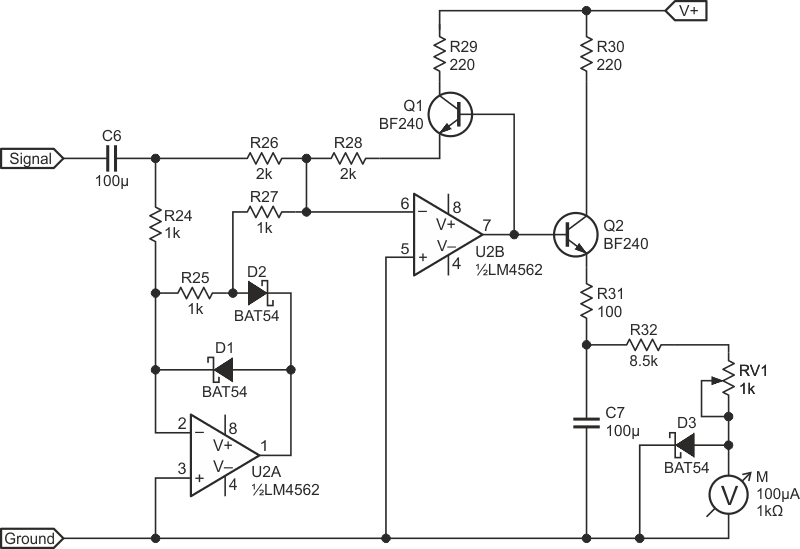Figure 5. A diagram showing the peak detector.

It has three features that secure wide bandwidth:

• The resistor values are much lower than usual in these circuits
• Schottky diodes are used in the first stage
• Two emitter followers are used to minimize loading at the output of the second stage

The BAT54 diodes can be replaced by BAT85. D3 protects the meter from excessive current if the range switch is set incorrectly.

The BF240 devices used in the prototype could be replaced by other devices with very similar characteristics. Although it is an old device, it is still available and was used for FM radio RF stages, so other devices for that same service may be suitable. Keep in mind that general-purpose devices like BC547 and 2N3904 are not suitable.

Furthermore, R24 and R26 could be made from two 1k resistors, also, R32 can be made from 10k and 56k resistors in parallel. Its value is chosen so the preset resistor, adjusted to read 1 V on the meter with 1 V RMS input at 1 kHz and the 3 dB attenuator applied, should be about halfway along its track.

A digital display can be connected in place of R32, RV1, and the meter, M.

## Power supply

The power supply circuitry is shown in Figure 6.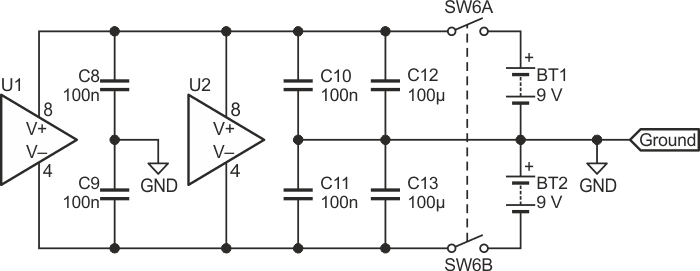Figure 6. Diagram showing the power supply.

The 100 nF capacitors should be mounted as close as possible to the IC pins on the supply side of the trace. If the trace went to the IC pin first and then to the capacitor, there would be unwanted inductance in series with the capacitor, and it doesn’t take much inductance to resonate with a 100 nF capacitor.

## Performance of the wideband analog meter project

Unless qualified (e.g., by ‘depends on layout’), the numbers are based on measured values of the prototype.

• Input resistance (all voltage ranges):
1 MΩ ±1%
• Input capacitance:
20 pF (depends on layout)
• Frequency response:
Flat from 20 Hz to 1 MHz: –1 dB at 10 Hz and 1.2 MHz.
• Voltage ranges:
1–10–100 mV–1–10–100 V; The accuracy depends on how close the range switch and attenuator resistors are to their correct values.
• DC output:
With 1 V RMS at 1 kHz applied at the voltage input and the range switch set to 1 V, with the 3 dB attenuator in the circuit, the DC output voltage at the junction of R29 and R30 should be within the range 0.95 V to 1.05 V. If it is not, adjust the value of R16 slightly (not to correct a LARGE error).
• Current ranges:
10–100 mA–1 A. The accuracy depends on the accuracy of the range-switching and how close the current sensing resistor R1 is to the correct value.
• DC output:
With 1 A RMS at 1 kHz applied at the Current input and the range switch set to 100 mV, with the 3 dB attenuator in the circuit, the DC output voltage at the junction of R29 and R30 should be within the range 0.95 V to 1.05 V. If it is not, adjust the value of R1 slightly (not to correct a LARGE error).

You can produce 1 A by using an audio amplifier with an 8 Ω ±1% resistor (dissipating only 0.125 W, so a ½ W part is okay) in series with the output and the current input. Adjust the input signal level to get 8 V RMS across the 8 Ω resistor.

The frequency responses at each range setting are shown in Figures 7, 8, 9, and 10.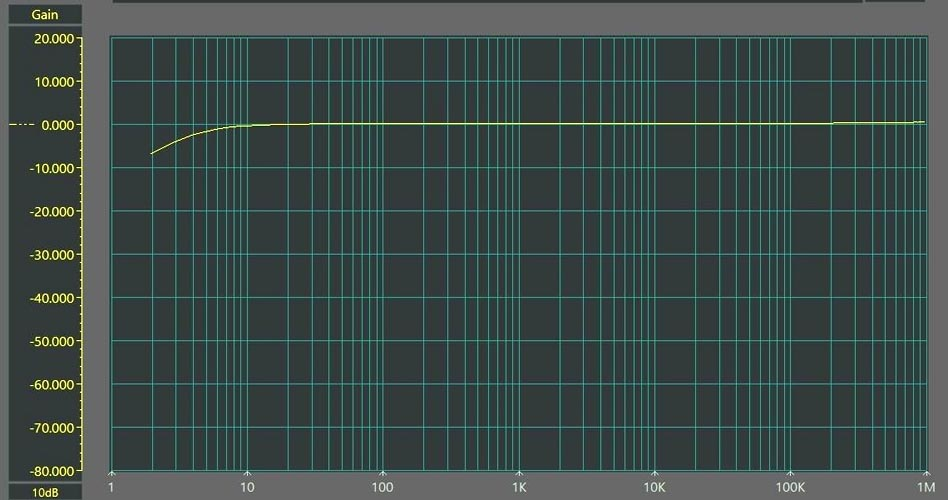Figure 7. Frequency response with 1 V input on 1 V range.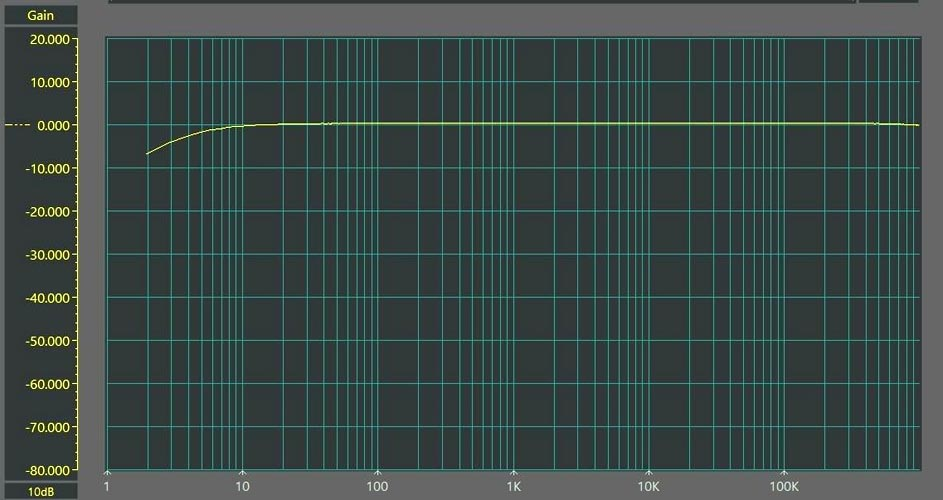Figure 8. Frequency response with 100 mV input on 100 mV range.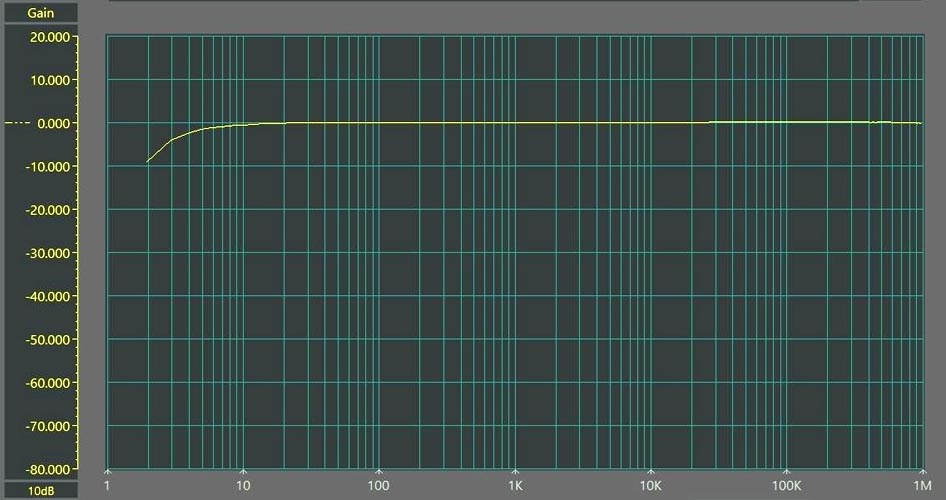Figure 9. Frequency response with 10 mV input on 10 mV range/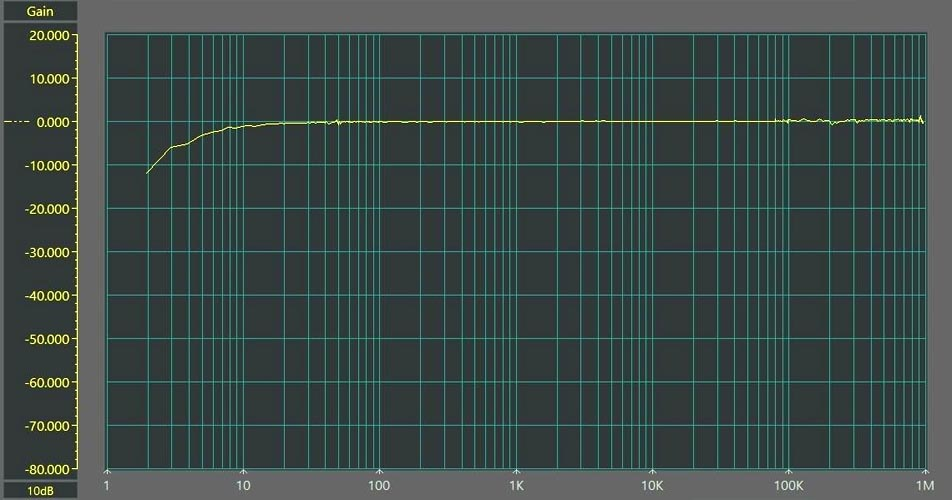Figure 10. Frequency response with 1 mV input on 1 mV range.

The responses at 1.2 MHz are out of range of the sweeper, so they were measured with a signal generator. In this range, a small amount of noise on the trace is to be expected due to the small input and high gain. Figure 11 shows the response of the current meter at 100 mA.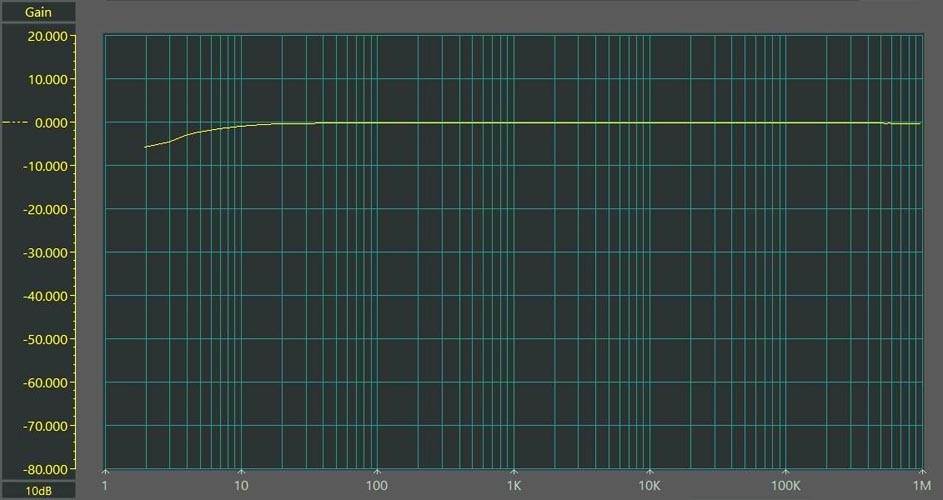Figure 11. Response of the current meter at 100 mA.

## Optional external filters

External filters should have an input resistance of 10 kΩ or greater and an output resistance of 1 kΩ or less.

## Construction tips

To keep unwanted RF emissions out of the meter, a metal or conductive-plastic enclosure is needed. It may be necessary to put ferrite beads at all inputs and outputs as close to the connector as possible. A very low-value capacitor, such as 10 pF, might be required between the inputs of each op amp.

## Possible design extensions

Several interesting extensions could be added to this design to provide additional features, including:

• An alternative, or selectable, true-RMS detector with 200 kHz bandwidth, using the reasonably-priced AD736 device
• A 22.4 Hz to 22.4 kHz standard unweighted audio noise filter
• An accurate A-weighting filter